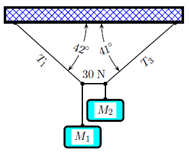Problem: In the figure below the left-hand cable has a tension T1 and makes an angle of 42° with the horizontal. The right-hand cable has a tension T3 and makes an angle of 41° with the horizontal. A W1 weight is on the left and a W2 weight is on the right. The cable connecting the two weights has a tension 30 N and is horizontal. The acceleration of gravity is 9.8 m/s2. Determine the mass M2. 1. 6.6 2. 5.32 3. 7.58 4. 3.77 5. 2.66 6. 4.7 7. 9.73 8. 4.96 9. 4.53 10. 8.27

FREE Expert Solution
86% (285 ratings)
Problem Details

In the figure below the left-hand cable has a tension T1 and makes an angle of 42° with the horizontal. The right-hand cable has a tension T3 and makes an angle of 41° with the horizontal. A W1 weight is on the left and a W2 weight is on the right. The cable connecting the two weights has a tension 30 N and is horizontal. The acceleration of gravity is 9.8 m/s2. Determine the mass M2.

1. 6.6

2. 5.32

3. 7.58

4. 3.77

5. 2.66

6. 4.7

7. 9.73

8. 4.96

9. 4.53

10. 8.27